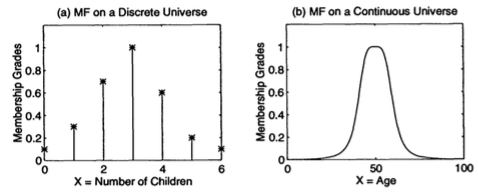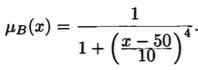### Introduction

A classical set (Also known as ordinary set, crisp set, nonfuzzy set or just set) is a set with a crisp boundary. For example, a classical set A of a real number greater than 6 can be expressed as

`A = { x | x > 6 }                                E.q. 1`
where there is a clear, unambiguous boundary 6 such that if x is greater than this number, then x belongs to the set A; otherwise x does not belong to the set. Although classical sets are suitable for various applications and have proven to be an important tool for mathematics and computer science, they do not reflect the nature of human concepts and thoughts, which tend to be abstract and imprecise. As an illustration, mathematically we can express the set of tall persons as a collection of persons whose height is more than 6 ft; this is the set denoted by E.q. 1, if we let A = "tall person" and x = "height". Yet this is an unnatural and inadequate way of representing our usual concept of "tall person". For one thing, the dichotomous nature of the classical set would classify a person 6.0001 ft tall as a tall person, but not a person 5.999 ft tall. This distinction is intuitively unreasonable. The flaw comes from the sharp transition between inclusion and exclusion in a set.

In contrast to a classical set, a fuzzy set, as the name implies, is a set without a crisp boundary. That is, the transition from "belong to a set" to "not belong to a set" is gradual, and this smooth transition is characterized by membership functions that give fuzzy sets flexibility in modeling commonly used linguistic expressions, such as "the water is hot" or "the temperature is high". As Zadeh pointed out in 1965 in his seminal paper entitled "Fuzzy Sets", such imprecisely defined sets or classes "play an important role in human thinking particularly in the domains of patter recognition, communication of information, and abstraction". Note that the fuzziness does not come from the randomness of constituent members of the sets, but from the uncertain and imprecise nature of abstract thoughts and concepts.

### Basic Definitions and Terminology

Let X be a space of objects and x be a generic element of X. A classical set A, A ⊆ X, is defined as a collection of elements or objects x ∈ X, such that each x can either belong or not belong to the set A. By defining a characteristic function for each elements x in X, we can represent a classical set A by a set of ordered pairs (x, 0) or (x, 1), which indicates x ∉ A or x ∈ A, respectively.

Unlike the aforementioned conventional set, a fuzzy set expresses the degree to which an element belongs to a set. Hence the characteristic function of a fuzzy set is allowed to have values between 0 and 1, which denotes the degree of membership of an element in a given set.

#### Fuzzy sets and membership functions

If X is a collection of objects denoted generically by x, then a fuzzy set A in X is defined as a set of ordered pairs:

`A = { (x, μA(x)) | x ∈ X }`
where μA(x) is called the membership function (MF) for the fuzzy set A. The MF maps each element of X to a membership grade between 0 and 1.

Obviously, the definition of a fuzzy set is a simple extension of the definition of a classical set in which the characteristic function is permitted to have any values between 0 and 1. If the value of the membership function μA(x) is restricted to either 0 or 1, then A is reduced to a classical set and μA(x) is the characteristic function of A.

Usually X is referred to as the universe of discourse, or simply the universe , and it may consist of discrete (ordered or nonordered) objects or continuous space.

#### Fuzzy sets with a discrete nonordered universe

Let X = {San Francisco, Boston, Los Angeles} be the set of cities one may choose to live in. The fuzzy set C = "desireable city to live in" may be described as follows:

`C = { (San Francisco, 0.9), (Boston, 0.8), (Los Angeles, 0.6) }`

Apparently the universe of discourse X is discrete and it contains nonordered objects - in this case, three big cities in the US. As one can see, the foregoing membership grades listed above are quite subjective; anyone can come up with three different but legitimate values to reflect his or her preference.

#### Fuzzy sets with a discrete ordered universeFigure 1: (a) A = "sensible number of children in a family"; (b): B = "about 50 years old."

Let X = { 0, 1, 2, 3, 4, 5, 6 } be the set of numbers of children a family may choose to have. Then the fuzzy set A = "sensible number of children in a family" may be described as follows:

`A = { (0, 0.1), (1, 0.3), (2, 0.7), (3, 1), (4, 0.7), (5, 0.3), (6, 0.1) }`

Here we have a discrete ordered universe X; the MF for the fuzzy set A is shown in Figure 1(a). Again, the membership grades of this fuzzy set are obviously subjective measures.

#### Fuzzy sets with a continuous universe

Let X = R+ be the set of possible ages for human beings. Then the fuzzy set B = "about 50 years old" may be expressed as

`B = { (x, μB(x)) | x ∈ X }`

whereThis is illustrated in Figure 1(b)

From the preceding examples, it is obvious that the construction of a fuzzy set depends on two things:

• The identification of a suitable universe of discourse
• The specification of an appropriate membership function.

The specification of membership functions is subjective, which means that the membership functions specified for the same concept (say, "sensible number of children in a family") by different persons may vary considerably. This subjectivity comes from individual differences in perceiving or expressing abstract concepts and has little to do with randomness. Therefore, the subjectivity and nonrandomness of fuzzy sets is the primary difference between the study of fuzzy sets and probability theory, which deals with objective treatment of random phenomena.